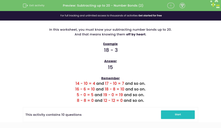# Practise Subtracting Bonds Up to 20

In this worksheet, students will be tested on their subtraction number bonds to 20.Key stage:  KS 1

Curriculum topic:   Number: Addition and Subtraction

Curriculum subtopic:   Add and Subtract to 20

Difficulty level:#### Worksheet Overview

In this activity, we need to know the subtracting number bonds up to 20.

We should know these off by heart.

The stars will remind you of the subtracting number bonds to 10:Can you see that the subtraction facts are the same as the addition facts but just the other way around?

For example, 10 - 1 = 9 would be 9 + 1 = 10. The numbers just get moved around, so if you know your adding number bonds, you will know your subtraction bonds!

Now, let's look at the number bond subtraction facts to 20.

20 - 0 = 20

20 - 1 = 19

20 - 2 = 18

20 - 3 = 17

20 - 4 = 16

20 - 5 = 15

20 - 6 = 16

20 - 7 = 17

20 - 8 = 18

20 - 9 = 19

20 - 10 = 10

Can you follow the pattern to complete all the subtraction number bonds to 20?

Example

20 - _ = 5

If you know the missing number in this subtraction number bond off by heart, then brilliant, just write in the answer.

If you are not sure, move it around into an addition number bond:

5 + _  = 20

What would go with 5 to make 20? It's 15. Remember, if you know your addition number bonds, you will know your subtraction number bonds!

Are you ready to have a go?### What is EdPlace?

We're your National Curriculum aligned online education content provider helping each child succeed in English, maths and science from year 1 to GCSE. With an EdPlace account you’ll be able to track and measure progress, helping each child achieve their best. We build confidence and attainment by personalising each child’s learning at a level that suits them.

Get started